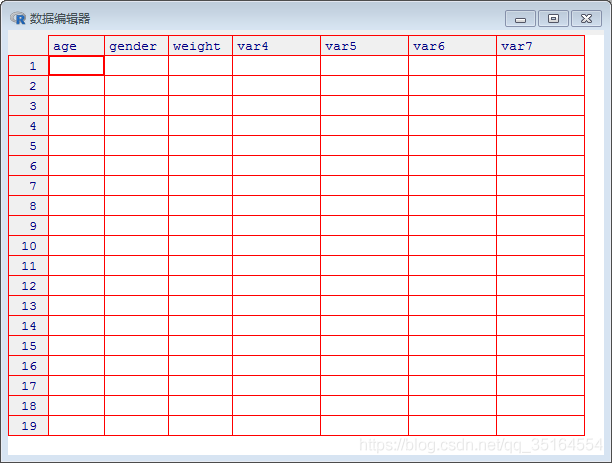# 数据分析基础｜R语言（数据集的创建）

+关注继续查看

## 创建数据集

### 数据结构

R语言中有许多用于存储数据的对象类型：标量、向量、矩阵、数组、数据框、列表等。

a <- 1
b <- "one"
c <- TRUER语言中用函数c()来创建向量的输入，向量输入的类型可以包含数值型、字符型（需要加引号）、布尔型（注意TRUE/FALSE都需要大写）

Vector_number <- c(1,2,3,4,5)
Vector_str <- c("one","two","three")
Vector_bool <- c(TRUE,FALSE,TRUE,TRUE)Vector <- c(1:5)> Vector_str[c(1,3)]
 "one"   "three"
> Vector_str
 "one"   "two"   "three"
> Vector_number
 1 2 3 4 5
> Vector_bool
  TRUE FALSE  TRUE  TRUEmatrix <- matrix(vector,nrow=number_of_rows,ncol=number_of_columns,byrow=logical_value,dimnames=list(char_vector_rownames,char_vector_colnames))Matrix <- matrix(1:20,nrow=4,ncol=5)
Mymatrix1 <- matrix(1:4,nrow=2,ncol=2,byrow=TRUE,dimname=list(c("r1","r2"),c("c1","c2")))
Mymatrix2 <- matrix(1:4,nrow=2,ncol=2,byrow=FALSE,dimname=list(c("r1","r2"),c("c1","c2")))> Matrix
[,1] [,2] [,3] [,4] [,5]
[1,]    1    5    9   13   17
[2,]    2    6   10   14   18
[3,]    3    7   11   15   19
[4,]    4    8   12   16   20
> Mymatrix1
c1 c2
r1  1  2
r2  3  4
> Mymatrix2
c1 c2
r1  1  3
r2  2  4array <- array(vector,dimensions,dimnames)Array <- array(1:18,c(3,2,3),dimnames=list(dim1,dim2,dim3))> Array
, , C1
B1 B2
A1  1  4
A2  2  5
A3  3  6
, , C2
B1 B2
A1  7 10
A2  8 11
A3  9 12
, , C3
B1 B2
A1 13 16
A2 14 17
A3 15 18mydata <- data.frame(col1,col2,col3,...)ID <- c(1,2,3,4)
name <- c("James","Durant","Curry","Harden")
number <- c(23,35,30,13)
patientdata <- data.frame(ID,name,number)> patientdata
ID   name number
1  1  James     23
2  2 Durant     35
3  3  Curry     30
4  4 Harden     13> patientdata
ID   name number
1  1  James     23
2  2 Durant     35
3  3  Curry     30
4  4 Harden     13
> patientdata[1:2]
ID   name
1  1  James
2  2 Durant
3  3  Curry
4  4 Harden
> patientdata[c("name","number")]
name number
1  James     23
2 Durant     35
3  Curry     30
4 Harden     13
> patientdata$name  James Durant Curry Harden Levels: Curry Durant Harden James$用来选取一个给定数据框中的某个特定变量。

> g <- "My First List"
> h <- c(25,26,18,39)
> j <- matrix(1:10,nrow=5)
> k <- c("one","two","three")
> mylist <- list(title=g,ages=h,j,k)
> mylist
$title  "My First List"$ages
 25 26 18 39
[]
[,1] [,2]
[1,]    1    6
[2,]    2    7
[3,]    3    8
[4,]    4    9
[5,]    5   10
[]
 "one"   "two"   "three"### 数据的输入

（1）创建一个空数据框（或矩阵），其中变量名和变量的模式需要与理想中的最终数据集保持一致。

（2）针对这个数据对象调用文本编辑器，输入你的数据，并将结果保存回次数据对象中。

> mydata <- data.frame(age=numeric(0),gender=character(0),weight=numeric(0))
> edit(mydata)mydataframe <- read.table(file,header=logical_value,sep="delimiter",row.names="name")grades <- read.table("studentgrades.csv",header=TRUE，sep=",",row.names="STUDENTID")install.packages("RODBC")
library(RODBC)
channel <- odbcConnectExcel("myfile.xls")
mydataframe <- sqlFetch(channel,"mysheet")
odbcClose(channel)|
1月前
|

r语言数据分析画数据相关性图热力图
r语言数据分析画数据相关性图热力图
27 1
|
4月前
|

R语言之处理大型数据集的策略
R语言之处理大型数据集的策略
75 4
|
5月前
|

88 0
|
6月前
|

R语言- data.table包加速大型数据集的加载和运算效率用法示例

112 0
|
7月前
|

103 0
|

Python 零基础入门数据分析实战之数据集应用

207 0|

ML之FE：利用【数据分析+数据处理】算法对国内某平台上海2020年6月份房价数据集【12+1】进行特征工程处理(史上最完整，建议收藏)——附录
ML之FE：利用【数据分析+数据处理】算法对国内某平台上海2020年6月份房价数据集【12+1】进行特征工程处理(史上最完整，建议收藏)——附录
112 0
|